## 3. 2. 4 Laplace Equation

One method typically used in simulation is the dual graph method. As an introductory example, the numerical solution of the Laplace equation is shown. In this case the functional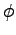is the gradient and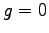.

For linear shape functions, the function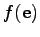along one edge can be written as(3.34)

The function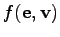denotes a shape function defined on the edge-skeleton of the cell complex. These functions have local support on one edge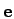and yield unity in the vertex. Consequently, the gradient is constant along the edge and, therefore, the evaluation point of the function as described in () does not play any role.(3.35)

The complete expression for the discretized Laplace equation yields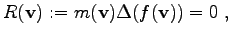(3.36)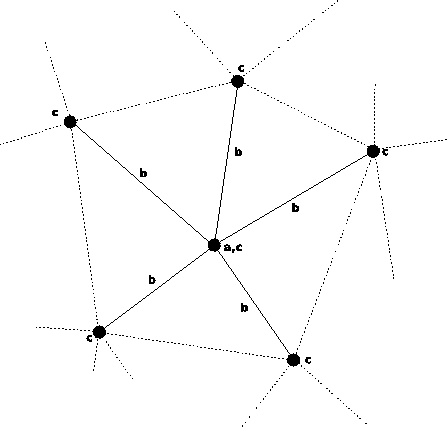Michael 2008-01-16